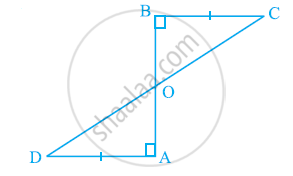# AD and BC are equal perpendiculars to a line segment AB (See the given figure). Show that CD bisects AB. - Mathematics

AD and BC are equal perpendiculars to a line segment AB (See the given figure). Show that CD bisects AB.#### Solution

In ΔBOC and ΔAOD,

∠BOC = ∠AOD (Vertically opposite angles)

∠CBO = ∠DAO (Each 90º)

∴ ΔBOC ≅ ΔAOD (AAS congruence rule)

∴ BO = AO (By CPCT)

⇒ CD bisects AB.

Concept: Criteria for Congruence of Triangles
Is there an error in this question or solution?
Chapter 7: Triangles - Exercise 7.1 [Page 119]

#### APPEARS IN

NCERT Class 9 Maths
Chapter 7 Triangles
Exercise 7.1 | Q 3 | Page 119
Share